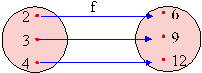Home MonkeyNotes Printable Notes Digital Library Study Guides Study Smart Parents Tips College Planning Test Prep Fun Zone Help / FAQ How to Cite New Title Request

 6.2 Functions Special relations : A and B are two non-empty sets. If each element of set the A is associated with exactly one element of set B, then this association is called a function from set A to set B. Note :- Set A is the domain Set B is the co-domain All the elements of set B need not have association The element of set B which are associated is the set "range" of the function. Thus the range is a sub-set of co-domain. For example, { ( 2, 9 ) , ( 3, 13 ) } is a function { ( 2, 5 ), ( 3, 9 ) } is not a function The element y Î B such that the function say 'f ' associates to the element x Î A is denoted by f ( x ) i.e. y = f ( x ) and y is called the 'f ' image of x or value of 'f ' at x. The element x is also called pre-image of y. Every element of A has a unique image but each element of B need not have an image of an element in A. There can be more than one element of A which has the same image in B. We denote the range of f : A ® B by f (A) Thus f ( A ) = { f ( x ) | x Î A } Þ f ( A ) Î B. The domain variable is often referred as the independent variable and the range variable is referred to as the dependent variable. Methods of representing a functionAn arrow diagram can be used. For example if f : x ® 3 x, x Î { 2, 3, 4 } then f can be shown as Index 6.1 Relations 6.2 Functions6.3 Variation Chapter 7
 Search: All Products Books Popular Music Classical Music Video DVD Toys & Games Electronics Software Tools & Hardware Outdoor Living Kitchen & Housewares Camera & Photo Cell Phones Keywords: